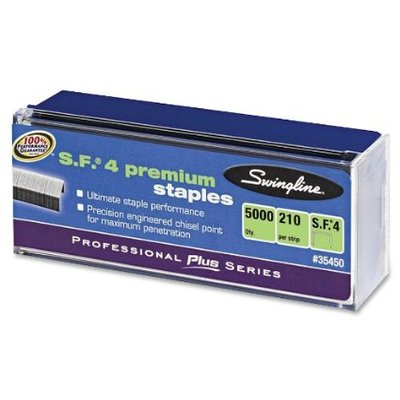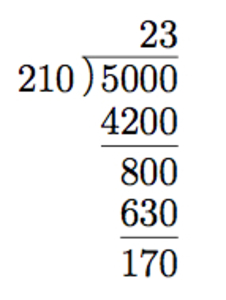# How many staples?

Alignments to Content Standards: 6.NS.B.2Can you find an inconsistency in the information on this box of staples? Explain.

## IM Commentary

The goal of this task is to perform long division with remainder in a context. The teacher will likely need to provide multiple levels of support on this question. First there is a lot of information on the box of staples and the relevant information for the task is the total quantity of staples and number of staples in each strip. Secondly, it might be helpful to have some packages of staples on hand so that students can see how they are packaged, in particular that the strips come in pairs and that there are usually 24 strips per package. With this extra information, 5000 should be 24 $\times$ 210, the number of strips times the number of staples per strip. Lastly, we do not know whether or not the reported numbers are exact or rounded. While the numbers can not be correct if they are exact, they can be correct if they are rounded.

In addition to the multiplication argument indicated above, students can use long division to check that 5000 staples cannot be divided into equal groups of 210 staples. This argument is presented first as it does not require knowledge of how many strips are in the package: in fact, it leads us to the conclusion that there are most likely 24 strips of staples. Another argument (presented second), examining the factors of 5000 and of 210, shows that 5000 staples can not be divided into groups of 210 without finding the quotient or remainder for 5000 $\div$ 210.

The closest number to 210 which is a divisor of 5000 is 200. While the 5000 staples could be divided evenly into 25 strips with 200 staples each, the packaging makes it more convenient to have 24 strips of staples. We can check that 5000 $\div$ 24 = 208$\frac{1}{3}$. So 210 is a good estimate for the number of staples in each strip but the strips must be of different lengths if there are exactly 5000 total staples in the package. Students might enjoy dividing up some strips and counting the staples to see if there are actually 210 staples in each strip.

## Solutions

Solution: Long Division

We divide the 5000 staples total by the number of staples in each strip, 210:This shows that we can make 23 strips of 210 staples, with 170 staples left over. We cannot divide the 5000 staples evenly into strips of 210. If we try a smaller number of staples, such as 208, we find that we can make 24 strips with 208 staples, leaving 8 left over:It is possible that the box contains some strips (sixteen) with 208 staples and some strips (eight) with 209. This would be reasonable as then there are approximately 210 staples in each strip. It is also possible that there are 24 strips of 210 staples, leaving 40 staples more than the 5000 stated on the package. While they cannot be exact, the numbers on the package of staples could be accurate within rounding error.

Solution: Factoring numbers

If there are 210 staples in each strip of staples and 5000 staples total then 5000 is a multiple of 210. Since 3 and 7 are factors of 210, this would mean that 3 and 7 are factors of 5000. But the only prime factors of 5000 are 5 and 2:$$5000 = 2 \times 2 \times 2 \times 5 \times 5 \times 5 \times 5.$$ This means that there cannot be 210 staples in each strip and 5000 staples total in the package.

Solution: Multiplication

If we are able to open the box of staples and look inside, we see that there are 24 strips of staples which come in pairs. If there are 210 staples per strip then the package would contain $$24 \times 210 = 5040$$ staples. So this means that either there are not exactly 210 staples in each strip or there are not exactly 5000 staples in the package.